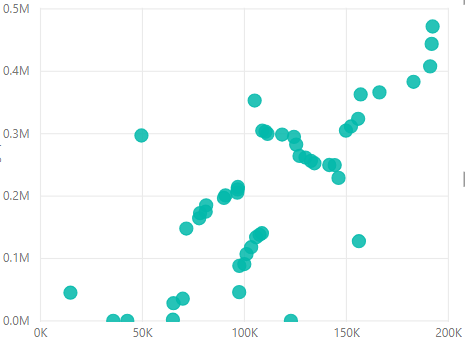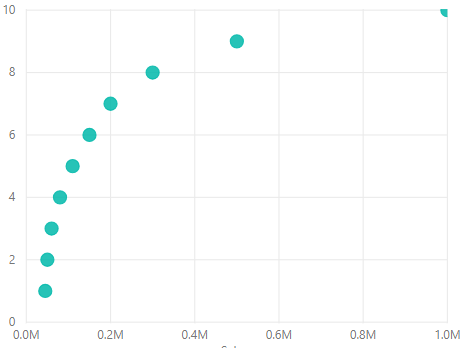# Multiple Linear Regression With Python

Simple Linear Regression

Regression is a machine learning model which we can use to predict values by using previously observed data. In simple linear regression, we had to use only one independent variable for the prediction. but in the real world often a dependent variable is dependent upon several variables. so we can't use the simple linear regression model in those cases.

This is where the multiple linear regression model comes in. it allows you to use multiple independent variables to predict the dependent variable. The formula for the multiple linear regression is given below.

$$y = \beta_0 + \beta_1 x_1 + \beta_2 x_2 + ... \beta_n x_n$$

Here $$\beta_0$$ is the constant and $$\beta_1 - n$$ are the coefficients that the model will have to figure out throughout the learning process.

The data set that we are going to use in this example is a data set which contains the spending and profit data of some companies. we can use multiple linear regression to identify the correlation of the spending to the profit and predict for a new value set. you can download the data set from here.

let's start by importing the required modules

import numpy as np
import pandas as pd
from sklearn.preprocessing import OneHotEncoder
from sklearn.compose import ColumnTransformer
from sklearn.model_selection import train_test_split
from sklearn.linear_model import LinearRegression
import statsmodels.formula.api as s


Now we are ready to read our data set and clean it up to proceed further. and we will also split the data set so we can feed the data to the model right away.

dataset = pd.read_csv('startups.csv')

X = dataset.iloc[:, :-1].values
y = dataset.iloc[:, 4].values

# Encoding categories
ct = ColumnTransformer(
[('one_hot_encoder', OneHotEncoder(), )],
remainder='passthrough',
)

X = ct.fit_transform(X)

# Removing the extra dummy variable
X = X[:, 1:]

# Splitting the set
X_train, X_test, y_train, y_test = train_test_split(X, y, test_size = 0.2)


Okay. with this done, we have split the data set and can be used to feed to our regression model. In Python for regression, we can use the scikit-learn library, let's do in now.

# Create the regression model
regressor = LinearRegression()
# Fit the model for training data
regressor.fit(X_train, y_train)
# Predict for the test data
y_pred = regressor.predict(X_test)


Done. so now you can compare y_pred with y_test to see how our regression model has performed.

In order to use multiple linear regression on a data set. we have to make some assumptions about the data set. these assumptions must be true before applying the regression model on the data set.

First one is that the independent variables and the dependent variable must be linearly correlated. meaning the relationship of each independent variable must be a linear one. this can be tested using scatter plots. See the example Linear relationship (Left) and the Non-linear relationship visualized using scatter chartsThe second assumption we have to make is that the residuals of the regression should follow a normal distribution. Residuals are the error terms or the difference between observed and predicted values of the dependent variable. A histogram of the residuals can be used to check that they are normally distributed. Additionally, you can use a P-P Plot to see the normality.

The third assumption is that homoscedasticity. If you take independent values and residuals, there should be no clear pattern in the distribution.

The fourth assumption is that the residuals must not be correlated

The fifth assumption is that the Independent variables should not be correlated too much that those relationships can affect the model.